# A Billion Bits of the Center Column of the Rule 30 Cellular Automaton

The center column of the rule 30 cellular automaton over a billion steps of evolution

The resource contains a byte array of the data. Each byte gives 8 values from the center column, with the highest-order bit in each byte representing the value that appears first in the center column.

## Examples

### Basic Examples

Retrieve the sequence packed into bytes:

 In:=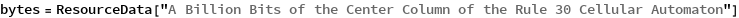Out=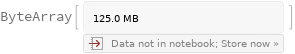Unpack the sequence as bits (this can take some time):

 In:=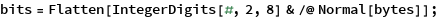The sequence contains a billion bits:

 In:=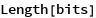Out=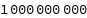It uses about 8 GB of memory:

 In:=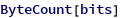Out=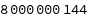Show the first 100 bits:

 In:=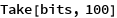Out=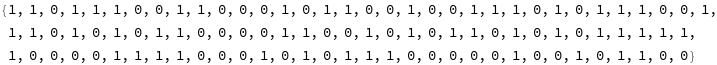Compare with a direct computation:

 In:=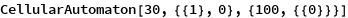Out=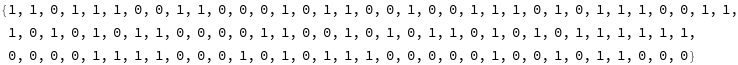Count the total number of 1s and 0s in the full sequence:

 In:=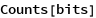Out=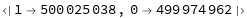Count the number of occurrences of possible 2-grams:

 In:=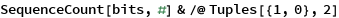Out=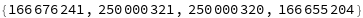Compute the running excess of 1s over 0s:

 In:=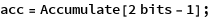Find the extreme values:

 In:=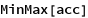Out=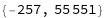Plot the running excess:

 In:=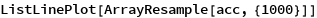Out=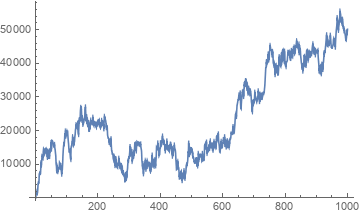Wolfram Research, "A Billion Bits of the Center Column of the Rule 30 Cellular Automaton" from the Wolfram Data Repository (2019)